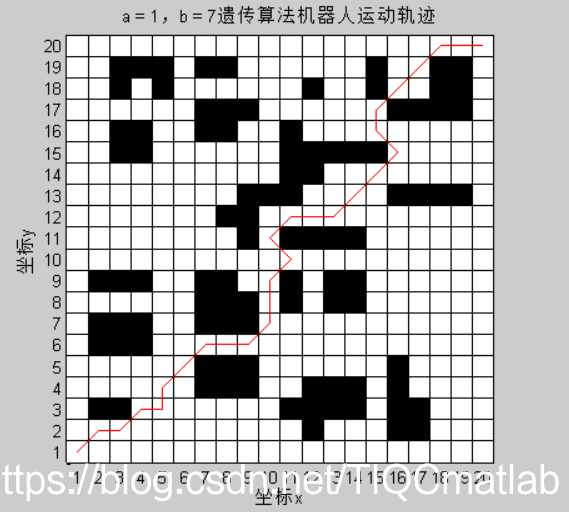# 【路径规划】基于matalb遗传算法机器人栅格地图路径规划【含Matlab源码 022期】

1、种群初始化

2、选择

3、交叉

4、变异

5、适应度计算

## 二、源代码

```c % 基于遗传算法的栅格法机器人路径规划 clc; clear all close all; % 输入数据,即栅格地图 G= [0 0 0 0 0 0 0 0 0 0 0 0 0 0 0 0 0 0 0 0; 0 0 0 0 0 0 0 0 0 0 0 1 0 0 0 1 1 0 0 0; 0 1 1 0 0 0 0 0 0 0 1 1 1 1 0 1 1 0 0 0; 0 0 0 0 0 0 1 1 1 0 0 1 1 1 0 1 0 0 0 0; 0 0 0 0 0 0 1 1 1 0 0 0 0 0 0 1 0 0 0 0; 0 1 1 1 0 0 0 0 0 0 0 0 0 0 0 0 0 0 0 0; 0 1 1 1 0 0 1 1 1 0 0 0 0 0 0 0 0 0 0 0; 0 0 0 0 0 0 1 1 1 0 1 0 1 1 0 0 0 0 0 0; 0 1 1 1 0 0 1 1 0 0 1 0 1 1 0 0 0 0 0 0; 0 0 0 0 0 0 0 0 0 0 0 0 0 0 0 0 0 0 0 0; 0 0 0 0 0 0 0 0 1 0 1 1 1 1 0 0 0 0 0 0; 0 0 0 0 0 0 0 1 1 0 0 0 0 0 0 0 0 0 0 0; 0 0 0 0 0 0 0 0 1 1 1 0 0 0 0 1 1 1 1 0; 0 0 0 0 0 0 0 0 0 0 1 1 0 0 0 0 0 0 0 0; 0 0 1 1 0 0 0 0 0 0 1 1 1 1 1 0 0 0 0 0; 0 0 1 1 0 0 1 1 0 0 1 0 0 0 0 0 0 0 0 0; 0 0 0 0 0 0 1 1 1 0 0 0 0 0 0 1 1 1 1 0; 0 0 1 0 1 0 0 0 0 0 0 1 0 0 1 0 0 1 1 0; 0 0 1 1 1 0 1 1 0 0 0 0 0 0 1 0 0 1 1 0; 0 0 0 0 0 0 0 0 0 0 0 0 0 0 0 0 0 0 0 0]; ​ p_start = 0; % 起始序号 p_end =399; % 终止序号 NP = 200; % 种群数量 max_gen = 2000; % 最大进化代数 pc = 0.5; % 交叉概率 pm = 0.5; % 变异概率 a = 1; % 路径长度比重 b = 7; % 路径顺滑度比重 %init_path = []; z = 1; new_pop1 = {}; % 元包类型路径 [y, x] = size(G); % 起点所在列（从左到右编号1.2.3...） xs = mod(p_start, x) + 1; % 起点所在行（从上到下编号行1.2.3...） ys = fix(p_start / x) + 1; % 终点所在列、行 xe = mod(p_end, x) + 1; ye = fix(p_end / x) + 1; ​ % 种群初始化step1，必经节点,从起始点所在行开始往上，在每行中挑选一个自由栅格，构成必经节点 pass_num = ye - ys + 1; pop = zeros(NP, pass_num); min_value=1000; for i = 1 : NP pop(i, 1) = p_start; j = 1; % 除去起点和终点 for yk = ys+1 : ye-1 j = j + 1; % 每一行的可行点 can = []; for xk = 1 : x % 栅格序号 no = (xk - 1) + (yk - 1) * x; if G(yk, xk) == 0 % 把点加入can矩阵中 can = [can no]; end end can_num = length(can); % 产生随机整数 index = randi(can_num); % 为每一行加一个可行点 pop(i, j) = can(index); end pop(i, end) = p_end; %pop % 种群初始化step2将上述必经节点联结成无间断路径 single_new_pop = generate_continuous_path(pop(i, :), G, x); %init_path = [init_path, single_new_pop]; if ~isempty(single_new_pop) new_pop1(z, 1) = {single_new_pop}; z = z + 1; end end ​ % 计算初始化种群的适应度 % 计算路径长度 path_value = cal_path_value(new_pop1, x); % 计算路径平滑度 path_smooth = cal_path_smooth(new_pop1, x); fit_value = a .* path_value .^ -1 + b .* path_smooth .^ -1; ​ mean_path_value = zeros(1, max_gen); min_path_value = zeros(1, max_gen); % 循环迭代操作 for i = 1 : max_gen % 选择操作 new_pop2 = selection(new_pop1, fit_value); % 交叉操作 new_pop2 = crossover(new_pop2, pc); % 变异操作 new_pop2 = mutation(new_pop2, pm, G, x); % 更新种群 new_pop1 = new_pop2; % 计算适应度值 % 计算路径长度 path_value = cal_path_value(new_pop1, x); % 计算路径平滑度 path_smooth = cal_path_smooth(new_pop1, x); fit_value = a .* path_value .^ -1 + b .* path_smooth .^ -1; mean_path_value(1, i) = mean(path_value); [~, m] = max(fit_value); if min(path_value)<min_value min_value=min(path_value); min_path_value(1, i) = min(path_value); else min_path_value(1, i) = min_value; end end % 画每次迭代平均路径长度和最优路径长度图 figure(1) plot(1:max_gen, mean_path_value, 'r') hold on; title(['a = ', num2str(a)', '，b = ',num2str(b)','的优化曲线图']); xlabel('迭代次数'); ylabel('路径长度'); plot(1:max_gen, min_path_value, 'b') legend('平均路径长度', '最优路径长度'); min_path_value(1, end) % 在地图上画路径 [~, min_index] = max(fit_value); min_path = new_pop1{min_index, 1}; figure(2) hold on; title(['a = ', num2str(a)', '，b = ',num2str(b)','遗传算法机器人运动轨迹']); xlabel('坐标x'); ylabel('坐标y'); DrawMap(G); [~, min_path_num] = size(min_path); for i = 1:min_path_num % 路径点所在列（从左到右编号1.2.3...） x_min_path(1, i) = mod(min_path(1, i), x) + 1; % 路径点所在行（从上到下编号行1.2.3...） y_min_path(1, i) = fix(min_path(1, i) / x) + 1; end hold on; plot(x_min_path, y_min_path, 'r')```

## 三、运行结果